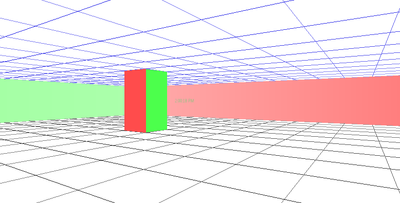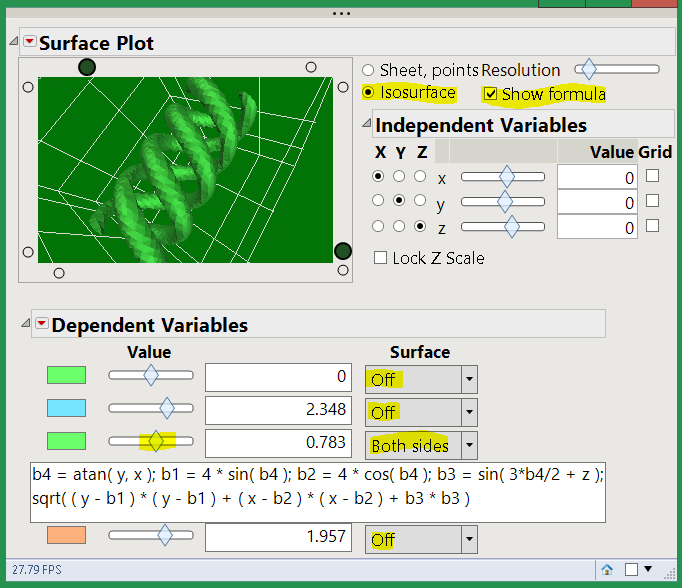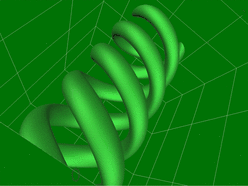Choose Language Hide Translation Bar
Three JSL Easter Eggs
• drawing program
• look in the scripting index, under MouseBox.  The sample script for SetClick is a tiny sketchpad.
• 15-puzzle
• while you are looking at MouseBox, check out the SetDropTrack script.  Classic game.
• maze
• Along with the other samples that ship with JMP, there is a set of 3D samples.  Look in Samples/Scripts/Scene3D.  Check out the RatMaze JSL sample.  (Read the 2nd paragraph of the comments before running it.)Article Labels

There are no labels assigned to this post.

Article Tags
1 CommentStaff (Retired)

One more. If you open the Surface Plot without any tables open, it displays a simple 2D sheet from a formula. If you select Isosurface and Show Formula and enable the 3rd formula and adjust the density, you'll get a triple helix.Surface Plot, no data table

JMP uses the formula to make a cube of data using the Points Resolution, then fits the Isosurface through the cloud of data using the slider value. The spinning GIF, below, was made with a high Points Resolution (right click on the slider to get a dialog to extend its range if you want to go really high.) Because of the N^3 number of points, higher resolutions take a while to get started and may rotate sluggishly after the points are generated.Triple Helix spinning in 3D

This formula is five statements long; the answer at each x,y,z point in the cube is the result of the final sqrt function. B4 is an angle in the x-y plane. B3 rotates on the z-axis, producing the twist.  The sqrt is forming the tubes. With any luck, you have a simpler formula. Or, more likely, you have a cloud of x,y,z samples.

The formula in the first slot is the 2D formula for the surface you see by default if you run Surface Plot with no data. The other two formulas are less interesting 3D formulas. You can overlay the 3D formulas and make them transparent (right-click ON the object to get the color/transparency dialog.) You can toss in the 2D formula too, but it has no Z in the formula so don't expect too much.

``````Surface Plot(
Show Surface( "Off" ),
Show Surface3( "Both sides" ),
Show formula( 1 ),
Mode( "Isosurface" ),
Resolution( 36 ),
Surface Alpha3( 1 ),
Mesh Color3( "Dark Green" ),
Surface Color3( "Light Green" ),

SetXVariable( x ),
SetYVariable( y ),
Iso Value( 2, 0.783 ),
Frame3D(
Set Graph Size( 335, 226 ),
Set Spin(
-0.0031,
0.0016,
0.79,
0.14
),
Set Wall Color( -5592405 ),
Set Walls( 0 ),
set light active( 2, 0 ),
set light position( 2, 8, -10, 0 ),
set light active( 6, 1 ),
set light color( 6, 33, 80, 36 ),
set light position( 6, 10, -8, 0 ),
set light color( 1, 35, 77, 34 ),
set light position( 1, -6.25, 10, 0 ),
Set Rotation( -84, 16, 103 ),
Set Line Scale( 0.1 ),
Set View Zoom( 0.6655 ),
Set View Perspective( 1.7631 ),
Background Color( 2, 115, 7 )
)
)``````

For the animated GIF I was experimenting with VLC to capture the screen as JMP rotated the isosurface. I think there may also be an addin to help with animated gifs.